# QRectF#

The `QRectF` class defines a finite rectangle in the plane using floating point precision. More

## Synopsis#

### Functions#

Note

This documentation may contain snippets that were automatically translated from C++ to Python. We always welcome contributions to the snippet translation. If you see an issue with the translation, you can also let us know by creating a ticket on https:/bugreports.qt.io/projects/PYSIDE

## Detailed Description#

Warning

This section contains snippets that were automatically translated from C++ to Python and may contain errors.

A rectangle is normally expressed as a top-left corner and a size. The size (width and height) of a `QRectF` is always equivalent to the mathematical rectangle that forms the basis for its rendering.

A `QRectF` can be constructed with a set of left, top, width and height coordinates, or from a `QPointF` and a `QSizeF` . The following code creates two identical rectangles.

```r1 = QRectF(100.0, 200.1, 11.2, 16.3)
r2 = QRectF(QPointF(100.0, 200.1), QSizeF(11.2, 16.3))
```

There is also a third constructor creating a `QRectF` from a `QRect` , and a corresponding `toRect()` function that returns a `QRect` object based on the values of this rectangle (note that the coordinates in the returned rectangle are rounded to the nearest integer).

The `QRectF` class provides a collection of functions that return the various rectangle coordinates, and enable manipulation of these. `QRectF` also provides functions to move the rectangle relative to the various coordinates. In addition there is a `moveTo()` function that moves the rectangle, leaving its top left corner at the given coordinates. Alternatively, the `translate()` function moves the rectangle the given offset relative to the current position, and the `translated()` function returns a translated copy of this rectangle.

The `size()` function returns the rectangle’s dimensions as a `QSizeF` . The dimensions can also be retrieved separately using the `width()` and `height()` functions. To manipulate the dimensions use the `setSize()` , `setWidth()` or `setHeight()` functions. Alternatively, the size can be changed by applying either of the functions setting the rectangle coordinates, for example, `setBottom()` or `setRight()` .

The `contains()` function tells whether a given point is inside the rectangle or not, and the `intersects()` function returns `true` if this rectangle intersects with a given rectangle (otherwise false). The `QRectF` class also provides the `intersected()` function which returns the intersection rectangle, and the `united()` function which returns the rectangle that encloses the given rectangle and this: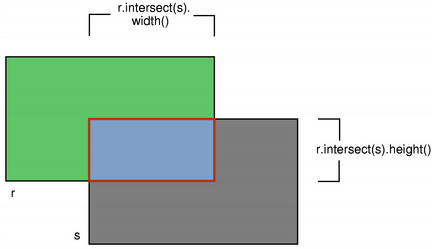`intersected()` `united()`

The `isEmpty()` function returns `true` if the rectangle’s width or height is less than, or equal to, 0. Note that an empty rectangle is not valid: The `isValid()` function returns `true` if both width and height is larger than 0. A null rectangle ( `isNull()` == true) on the other hand, has both width and height set to 0.

Note that due to the way `QRect` and `QRectF` are defined, an empty `QRectF` is defined in essentially the same way as `QRect` .

Finally, `QRectF` objects can be streamed as well as compared.

### Rendering#

When using an `anti-aliased` painter, the boundary line of a `QRectF` will be rendered symmetrically on both sides of the mathematical rectangle’s boundary line. But when using an aliased painter (the default) other rules apply.

Then, when rendering with a one pixel wide pen the `QRectF` ‘s boundary line will be rendered to the right and below the mathematical rectangle’s boundary line.

When rendering with a two pixels wide pen the boundary line will be split in the middle by the mathematical rectangle. This will be the case whenever the pen is set to an even number of pixels, while rendering with a pen with an odd number of pixels, the spare pixel will be rendered to the right and below the mathematical rectangle as in the one pixel case.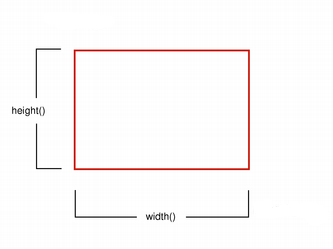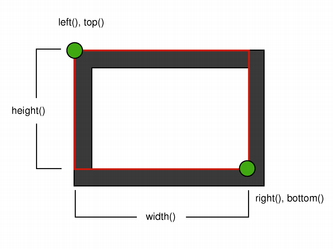Logical representation One pixel wide pen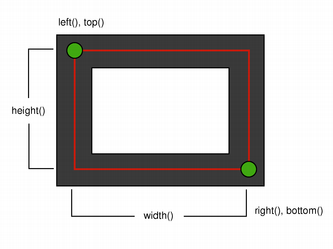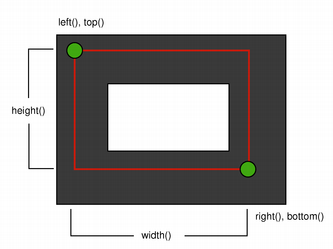Two pixel wide pen Three pixel wide pen

### Coordinates#

The `QRectF` class provides a collection of functions that return the various rectangle coordinates, and enable manipulation of these. `QRectF` also provides functions to move the rectangle relative to the various coordinates.

For example: the `bottom()` , `setBottom()` and `moveBottom()` functions: `bottom()` returns the y-coordinate of the rectangle’s bottom edge, `setBottom()` sets the bottom edge of the rectangle to the given y coordinate (it may change the height, but will never change the rectangle’s top edge) and `moveBottom()` moves the entire rectangle vertically, leaving the rectangle’s bottom edge at the given y coordinate and its size unchanged.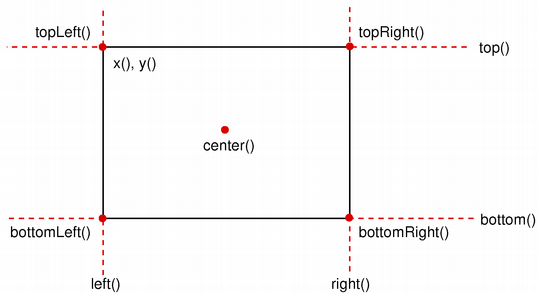It is also possible to add offsets to this rectangle’s coordinates using the `adjust()` function, as well as retrieve a new rectangle based on adjustments of the original one using the `adjusted()` function. If either of the width and height is negative, use the `normalized()` function to retrieve a rectangle where the corners are swapped.

In addition, `QRectF` provides the `getCoords()` function which extracts the position of the rectangle’s top-left and bottom-right corner, and the `getRect()` function which extracts the rectangle’s top-left corner, width and height. Use the `setCoords()` and `setRect()` function to manipulate the rectangle’s coordinates and dimensions in one go.

`QRect` `QRegion`

class PySide6.QtCore.QRectF#

PySide6.QtCore.QRectF(topleft, bottomRight)

PySide6.QtCore.QRectF(topleft, size)

PySide6.QtCore.QRectF(rect)

PySide6.QtCore.QRectF(left, top, width, height)

Parameters:

Constructs a null rectangle.

Constructs a rectangle with the given `topLeft` and `bottomRight` corners.

Constructs a rectangle with the given `topLeft` corner and the given `size`.

Constructs a `QRectF` rectangle from the given `QRect` `rectangle`.

Note

This function, like `toRectF()` , preserves the `size()` of `rectangle`, not its `bottomRight()` corner.

Constructs a rectangle with (`x`, `y`) as its top-left corner and the given `width` and `height`. All parameters must be finite.

PySide6.QtCore.QRectF.__reduce__()#
Return type:

object

PySide6.QtCore.QRectF.__repr__()#
Return type:

object

Parameters:
• x1 – float

• y1 – float

• x2 – float

• y2 – float

Adds `dx1`, `dy1`, `dx2` and `dy2` respectively to the existing coordinates of the rectangle. All parameters must be finite.

Parameters:
• x1 – float

• y1 – float

• x2 – float

• y2 – float

Return type:

`PySide6.QtCore.QRectF`

Returns a new rectangle with `dx1`, `dy1`, `dx2` and `dy2` added respectively to the existing coordinates of this rectangle. All parameters must be finite.

PySide6.QtCore.QRectF.bottom()#
Return type:

float

Returns the y-coordinate of the rectangle’s bottom edge.

PySide6.QtCore.QRectF.bottomLeft()#
Return type:

`PySide6.QtCore.QPointF`

Returns the position of the rectangle’s bottom-left corner.

PySide6.QtCore.QRectF.bottomRight()#
Return type:

`PySide6.QtCore.QPointF`

Returns the position of the rectangle’s bottom-right corner.

PySide6.QtCore.QRectF.center()#
Return type:

`PySide6.QtCore.QPointF`

Returns the center point of the rectangle.

PySide6.QtCore.QRectF.contains(p)#
Parameters:
Return type:

bool

Returns `true` if the given `point` is inside or on the edge of the rectangle; otherwise returns `false`.

PySide6.QtCore.QRectF.contains(r)
Parameters:
Return type:

bool

Returns `true` if the given `rectangle` is inside this rectangle; otherwise returns `false`.

PySide6.QtCore.QRectF.contains(x, y)
Parameters:
• x – float

• y – float

Return type:

bool

Returns `true` if the point (`x`, `y`) is inside or on the edge of the rectangle; otherwise returns `false`.

PySide6.QtCore.QRectF.getCoords()#
Return type:

PyObject

Extracts the position of the rectangle’s top-left corner to *``x1`` and *``y1``, and the position of the bottom-right corner to *``x2`` and *``y2``.

PySide6.QtCore.QRectF.getRect()#
Return type:

PyObject

Extracts the position of the rectangle’s top-left corner to *``x`` and *``y``, and its dimensions to *``width`` and *``height``.

PySide6.QtCore.QRectF.height()#
Return type:

float

Returns the height of the rectangle.

PySide6.QtCore.QRectF.intersected(other)#
Parameters:
Return type:

`PySide6.QtCore.QRectF`

Returns the intersection of this rectangle and the given `rectangle`. Note that `r.intersected(s)` is equivalent to `r & s`.`intersects()` `united()` `operator&=()`

PySide6.QtCore.QRectF.intersects(r)#
Parameters:
Return type:

bool

Returns `true` if this rectangle intersects with the given `rectangle` (i.e. there is a non-empty area of overlap between them), otherwise returns `false`.

The intersection rectangle can be retrieved using the `intersected()` function.

PySide6.QtCore.QRectF.isEmpty()#
Return type:

bool

Returns `true` if the rectangle is empty, otherwise returns `false`.

An empty rectangle has `width()` <= 0 or `height()` <= 0. An empty rectangle is not valid (i.e., isEmpty() == ! `isValid()` ).

Use the `normalized()` function to retrieve a rectangle where the corners are swapped.

PySide6.QtCore.QRectF.isNull()#
Return type:

bool

Returns `true` if the rectangle is a null rectangle, otherwise returns `false`.

A null rectangle has both the width and the height set to 0. A null rectangle is also empty, and hence not valid.

PySide6.QtCore.QRectF.isValid()#
Return type:

bool

Returns `true` if the rectangle is valid, otherwise returns `false`.

A valid rectangle has a `width()` > 0 and `height()` > 0. Note that non-trivial operations like intersections are not defined for invalid rectangles. A valid rectangle is not empty (i.e., isValid() == ! `isEmpty()` ).

PySide6.QtCore.QRectF.left()#
Return type:

float

Returns the x-coordinate of the rectangle’s left edge. Equivalent to `x()` .

Parameters:
Return type:

`PySide6.QtCore.QRectF`

Returns a rectangle grown by the `margins`.

`operator+=()` `marginsRemoved()` `operator-=()`

PySide6.QtCore.QRectF.marginsRemoved(margins)#
Parameters:
Return type:

`PySide6.QtCore.QRectF`

Removes the `margins` from the rectangle, shrinking it.

`marginsAdded()` `operator+=()` `operator-=()`

PySide6.QtCore.QRectF.moveBottom(pos)#
Parameters:

pos – float

Moves the rectangle vertically, leaving the rectangle’s bottom edge at the given finite `y` coordinate. The rectangle’s size is unchanged.

PySide6.QtCore.QRectF.moveBottomLeft(p)#
Parameters:

Moves the rectangle, leaving the bottom-left corner at the given `position`. The rectangle’s size is unchanged.

PySide6.QtCore.QRectF.moveBottomRight(p)#
Parameters:

Moves the rectangle, leaving the bottom-right corner at the given `position`. The rectangle’s size is unchanged.

PySide6.QtCore.QRectF.moveCenter(p)#
Parameters:

Moves the rectangle, leaving the center point at the given `position`. The rectangle’s size is unchanged.

PySide6.QtCore.QRectF.moveLeft(pos)#
Parameters:

pos – float

Moves the rectangle horizontally, leaving the rectangle’s left edge at the given finite `x` coordinate. The rectangle’s size is unchanged.

PySide6.QtCore.QRectF.moveRight(pos)#
Parameters:

pos – float

Moves the rectangle horizontally, leaving the rectangle’s right edge at the given finite `x` coordinate. The rectangle’s size is unchanged.

PySide6.QtCore.QRectF.moveTo(x, y)#
Parameters:
• x – float

• y – float

Moves the rectangle, leaving the top-left corner at the given position (`x`, `y`). The rectangle’s size is unchanged. Both parameters must be finite.

PySide6.QtCore.QRectF.moveTo(p)
Parameters:

Moves the rectangle, leaving the top-left corner at the given `position`.

PySide6.QtCore.QRectF.moveTop(pos)#
Parameters:

pos – float

Moves the rectangle vertically, leaving the rectangle’s top line at the given finite `y` coordinate. The rectangle’s size is unchanged.

PySide6.QtCore.QRectF.moveTopLeft(p)#
Parameters:

Moves the rectangle, leaving the top-left corner at the given `position`. The rectangle’s size is unchanged.

PySide6.QtCore.QRectF.moveTopRight(p)#
Parameters:

Moves the rectangle, leaving the top-right corner at the given `position`. The rectangle’s size is unchanged.

PySide6.QtCore.QRectF.normalized()#
Return type:

`PySide6.QtCore.QRectF`

Returns a normalized rectangle; i.e., a rectangle that has a non-negative width and height.

If `width()` < 0 the function swaps the left and right corners, and it swaps the top and bottom corners if `height()` < 0.

PySide6.QtCore.QRectF.__ne__(r2)#
Parameters:
Return type:

bool

Returns `true` if the rectangles `r1` and `r2` are sufficiently different, otherwise returns `false`.

Warning

This function does not check for strict inequality; instead, it uses a fuzzy comparison to compare the rectangles’ coordinates.

PySide6.QtCore.QRectF.__and__(r)#
Parameters:
Return type:

`PySide6.QtCore.QRectF`

Returns the intersection of this rectangle and the given `rectangle`. Returns an empty rectangle if there is no intersection.

`operator&=()` `intersected()`

PySide6.QtCore.QRectF.__iand__(r)#
Parameters:
Return type:

`PySide6.QtCore.QRectF`

Intersects this rectangle with the given `rectangle`.

`intersected()` `operator&()`

Parameters:
Return type:

`PySide6.QtCore.QRectF`

Returns the `lhs` rectangle grown by the `rhs` margins.

Parameters:
Return type:

`PySide6.QtCore.QRectF`

Returns the `lhs` rectangle grown by the `rhs` margins.

Parameters:
Return type:

`PySide6.QtCore.QRectF`

Adds the `margins` to the rectangle, growing it.

`marginsAdded()` `marginsRemoved()` `operator-=()`

PySide6.QtCore.QRectF.__sub__(rhs)#
Parameters:
Return type:

`PySide6.QtCore.QRectF`

Returns the `lhs` rectangle shrunk by the `rhs` margins.

PySide6.QtCore.QRectF.__isub__(margins)#
Parameters:
Return type:

`PySide6.QtCore.QRectF`

Returns a rectangle shrunk by the `margins`.

`marginsRemoved()` `operator+=()` `marginsAdded()`

PySide6.QtCore.QRectF.__eq__(r2)#
Parameters:
Return type:

bool

Returns `true` if the rectangles `r1` and `r2` are approximately equal, otherwise returns `false`.

Warning

This function does not check for strict equality; instead, it uses a fuzzy comparison to compare the rectangles’ coordinates.

`qFuzzyCompare`

PySide6.QtCore.QRectF.__or__(r)#
Parameters:
Return type:

`PySide6.QtCore.QRectF`

Returns the bounding rectangle of this rectangle and the given `rectangle`.

`united()` `operator|=()`

PySide6.QtCore.QRectF.__ior__(r)#
Parameters:
Return type:

`PySide6.QtCore.QRectF`

Unites this rectangle with the given `rectangle`.

`united()` `operator|()`

PySide6.QtCore.QRectF.right()#
Return type:

float

Returns the x-coordinate of the rectangle’s right edge.

PySide6.QtCore.QRectF.setBottom(pos)#
Parameters:

pos – float

Sets the bottom edge of the rectangle to the given finite `y` coordinate. May change the height, but will never change the top edge of the rectangle.

PySide6.QtCore.QRectF.setBottomLeft(p)#
Parameters:

Set the bottom-left corner of the rectangle to the given `position`. May change the size, but will never change the top-right corner of the rectangle.

PySide6.QtCore.QRectF.setBottomRight(p)#
Parameters:

Set the bottom-right corner of the rectangle to the given `position`. May change the size, but will never change the top-left corner of the rectangle.

PySide6.QtCore.QRectF.setCoords(x1, y1, x2, y2)#
Parameters:
• x1 – float

• y1 – float

• x2 – float

• y2 – float

Sets the coordinates of the rectangle’s top-left corner to (`x1`, `y1`), and the coordinates of its bottom-right corner to (`x2`, `y2`). All parameters must be finite.

PySide6.QtCore.QRectF.setHeight(h)#
Parameters:

h – float

Sets the height of the rectangle to the given finite `height`. The bottom edge is changed, but not the top one.

PySide6.QtCore.QRectF.setLeft(pos)#
Parameters:

pos – float

Sets the left edge of the rectangle to the given finite `x` coordinate. May change the width, but will never change the right edge of the rectangle.

Equivalent to `setX()` .

PySide6.QtCore.QRectF.setRect(x, y, w, h)#
Parameters:
• x – float

• y – float

• w – float

• h – float

Sets the coordinates of the rectangle’s top-left corner to (`x`, `y`), and its size to the given `width` and `height`. All parameters must be finite.

PySide6.QtCore.QRectF.setRight(pos)#
Parameters:

pos – float

Sets the right edge of the rectangle to the given finite `x` coordinate. May change the width, but will never change the left edge of the rectangle.

PySide6.QtCore.QRectF.setSize(s)#
Parameters:

Sets the size of the rectangle to the given finite `size`. The top-left corner is not moved.

PySide6.QtCore.QRectF.setTop(pos)#
Parameters:

pos – float

Sets the top edge of the rectangle to the given finite `y` coordinate. May change the height, but will never change the bottom edge of the rectangle.

Equivalent to `setY()` .

PySide6.QtCore.QRectF.setTopLeft(p)#
Parameters:

Set the top-left corner of the rectangle to the given `position`. May change the size, but will never change the bottom-right corner of the rectangle.

PySide6.QtCore.QRectF.setTopRight(p)#
Parameters:

Set the top-right corner of the rectangle to the given `position`. May change the size, but will never change the bottom-left corner of the rectangle.

PySide6.QtCore.QRectF.setWidth(w)#
Parameters:

w – float

Sets the width of the rectangle to the given finite `width`. The right edge is changed, but not the left one.

PySide6.QtCore.QRectF.setX(pos)#
Parameters:

pos – float

Sets the left edge of the rectangle to the given finite `x` coordinate. May change the width, but will never change the right edge of the rectangle.

Equivalent to `setLeft()` .

PySide6.QtCore.QRectF.setY(pos)#
Parameters:

pos – float

Sets the top edge of the rectangle to the given finite `y` coordinate. May change the height, but will never change the bottom edge of the rectangle.

Equivalent to `setTop()` .

PySide6.QtCore.QRectF.size()#
Return type:

`PySide6.QtCore.QSizeF`

Returns the size of the rectangle.

PySide6.QtCore.QRectF.toAlignedRect()#
Return type:

`PySide6.QtCore.QRect`

Returns a `QRect` based on the values of this rectangle that is the smallest possible integer rectangle that completely contains this rectangle.

PySide6.QtCore.QRectF.toRect()#
Return type:

`PySide6.QtCore.QRect`

Returns a `QRect` based on the values of this rectangle. Note that the coordinates in the returned rectangle are rounded to the nearest integer.

`QRectF()` `toAlignedRect()` `toRectF()`

PySide6.QtCore.QRectF.top()#
Return type:

float

Returns the y-coordinate of the rectangle’s top edge. Equivalent to `y()` .

PySide6.QtCore.QRectF.topLeft()#
Return type:

`PySide6.QtCore.QPointF`

Returns the position of the rectangle’s top-left corner.

PySide6.QtCore.QRectF.topRight()#
Return type:

`PySide6.QtCore.QPointF`

Returns the position of the rectangle’s top-right corner.

PySide6.QtCore.QRectF.translate(p)#
Parameters:

Moves the rectangle `offset`. `x()` along the x axis and `offset`. `y()` along the y axis, relative to the current position.

PySide6.QtCore.QRectF.translate(dx, dy)
Parameters:
• dx – float

• dy – float

Moves the rectangle `dx` along the x-axis and `dy` along the y-axis, relative to the current position. Positive values move the rectangle to the right and downwards. Both parameters must be finite.

PySide6.QtCore.QRectF.translated(p)#
Parameters:
Return type:

`PySide6.QtCore.QRectF`

Returns a copy of the rectangle that is translated `offset`. `x()` along the x axis and `offset`. `y()` along the y axis, relative to the current position.

PySide6.QtCore.QRectF.translated(dx, dy)
Parameters:
• dx – float

• dy – float

Return type:

`PySide6.QtCore.QRectF`

Returns a copy of the rectangle that is translated `dx` along the x axis and `dy` along the y axis, relative to the current position. Positive values move the rectangle to the right and down. Both parameters must be finite.

PySide6.QtCore.QRectF.transposed()#
Return type:

`PySide6.QtCore.QRectF`

Warning

This section contains snippets that were automatically translated from C++ to Python and may contain errors.

Returns a copy of the rectangle that has its width and height exchanged:

```r = {1.5, 5.1, 4.2, 2.4}
r = r.transposed() # r == {1.5, 5.1, 2.4, 4.2}
```
PySide6.QtCore.QRectF.united(other)#
Parameters:
Return type:

`PySide6.QtCore.QRectF`

Returns the bounding rectangle of this rectangle and the given `rectangle`.PySide6.QtCore.QRectF.width()#
Return type:

float

Returns the width of the rectangle.

PySide6.QtCore.QRectF.x()#
Return type:

float

Returns the x-coordinate of the rectangle’s left edge. Equivalent to `left()` .

Returns the y-coordinate of the rectangle’s top edge. Equivalent to `top()` .